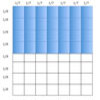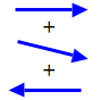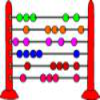Search by Topic

Filter by: Content type:
Age range:
Challenge level:

There are 7 NRICH Mathematical resources connected to Representing numbers, you may find related items under Numbers and the Number System.

Broad Topics > Numbers and the Number System > Representing numbersAge 7 to 14 Challenge Level:

I added together some of my neighbours' house numbers. Can you explain the patterns I noticed?Models in Mind

Age 3 to 14

This article looks at how models support mathematical thinking about numbers and the number systemGalley Division

Age 14 to 16 Challenge Level:

Can you explain how Galley Division works?Balance Power

Age 11 to 18 Challenge Level:

Using balancing scales what is the least number of weights needed to weigh all integer masses from 1 to 1000? Placing some of the weights in the same pan as the object how many are needed?A Story about Absolutely Nothing

Age 7 to 18

This article for the young and old talks about the origins of our number system and the important role zero has to play in it.Arrow Arithmetic 1

Age 14 to 16 Challenge Level:

The first part of an investigation into how to represent numbers using geometric transformations that ultimately leads us to discover numbers not on the number line.Fibonacci's Three Wishes 1

Age 7 to 14

First or two articles about Fibonacci, written for students.